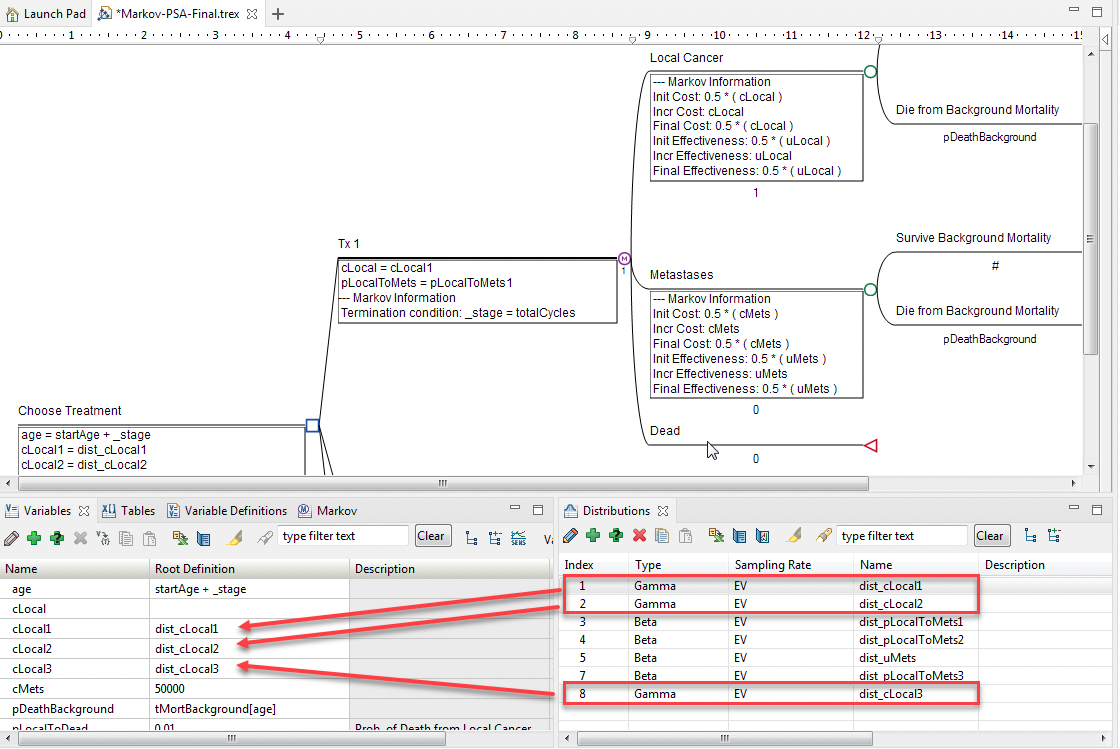# 33. Probabilistic Sensitivity Analysis on CE Models

This chapter describes how to run Probabilistic Sensitivity Analysis (PSA) to study the impact of parameter uncertainty on cost-effectiveness models. Before running PSA, you need to use distributions for some of your inputs, so samples can be drawn from those distributions to represent parameter uncertainty. This chapter will cover how to include those distributions in your model

Background

Probabilistic Sensitivity Analysis (PSA) studies the impact of parameter uncertainty on models. PSA is important because it helps us understand how confident we are in conclusions drawn from our model. In this chapter, we will examine running PSA on cost-effectiveness models.

When we run PSA, we calculate the model many times with different sets of inputs sampled from distributions that represent parameter uncertainty. We can then look at the set of results to understand the impact of that combined uncertainty. At a basic level, the higher percentage of calculations that confirm your base case, the more confidence you have in your conclusions.

Before running PSA, we must first add and reference parameter-level distributions for some of the model inputs. These distributions are then resampled for each calculation of the model.

PSA can be conducted on all model types supported by TreeAge Pro - Decision Tree, Markov, Partitioned Survival Analysis, Patient Simulation and Discrete Event Simulation.

We will use two models in this Chapter:

• Markov-PSA-PreSetup.trex prior to adding distributions

• Markov-PSA-Final.trex after adding the distributions

The “final” model is ready for PSA.PSA can be run on both cohort and simulation models. This chapter will focus mostly on cohort models, but the results for simulation models are presented in the same format. PSA on simulation models will be covered at the end of the Patient Level Simulation (Microsimulation) section.